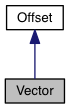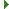PDG engine v0.9.5
Vector Class Reference

vector (magnitude and direction) within a 2D coordinate system. More...

Inheritance diagram for Vector:[legend]
Collaboration diagram for Vector:[legend]

## Public Member Functions

Vector ()

Vector (number x, number y)

Vector (number[] xy)

Vector (object xy)

number dotProduct (Vector vector)

number vectorAngle ()

number vectorLength ()Public Member Functions inherited from Offset
Offset ()

Offset (number x, number y)

Offset (number[] xy)

Offset (object xy)

Offset add (Offset offset)

Offset assign (Offset offset)

Offset div (Offset offset)

Offset dividedby (Offset offset)

boolean equals (Offset offset)

Offset minus (Offset offset)

Offset mul (Offset offset)

boolean notEquals (Offset offset)

Offset plus (Offset offset)

Offset sub (Offset offset)

Offset times (Offset offset)

## Public Attributes

number x

number yPublic Attributes inherited from Offset
number x

number y

## Detailed Description

vector (magnitude and direction) within a 2D coordinate system.

Point provides support for dealing with vectors as x, y floating point values in 2 dimensional space. A vector is similar to an Offset but can be treated as a magnitude and direction where an Offset can't. The PDG coordinate space starts with the top left corner of the drawing port at Point(0,0) and the bottom left corner at Point( width, height );

## Constructor & Destructor Documentation

 Vector ( )
 Vector ( number x, number y )
 Vector ( number[] xy )
 Vector ( object xy )

## Member Function Documentation

 number dotProduct ( Vector vector )
 number vectorAngle ( )
 number vectorLength ( )

## Member Data Documentation

 number x
 number y